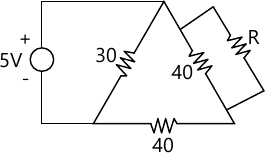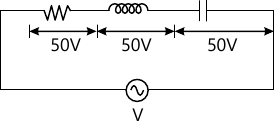MORE IN Basic Electrical Engg.
VTU First Year Engineering (P Cycle) (Semester 2)
Basic Electrical Engg.
December 2013
Total marks: --
Total time: --
INSTRUCTIONS
(1) Assume appropriate data and state your reasons
(2) Marks are given to the right of every question
(3) Draw neat diagrams wherever necessary

Choose the correct answer for the following :-
1 (a) (i) The polarity of voltage drop across is resistor is determine by ______
(A) the values of resistor
(B) the values of current
(C) direction of current in resistor
(D) the polarity of source
1 M
1 (a) (ii) Three resistor of 4?, 6? and 9? are connected in parallel in a network. Maximum power will be consumed by _____
(A) 4? resistor
(B) 6? resistor
(C) 9? resistor
(D) all resistor
1 M
1 (a) (iii) The magnitude of statically induced e.m.f. depends on
(A) the coil resistance
(B) the flux magnitude
(C) the rate of change of flux
(D) all of these
1 M
1 (a) (iv) The direction of induced e.m.f. in a conductor (or coil) can be determined by _____
(A) Work law
(B) Ampere's law
(C) Fleming's right hand rule
(D) Fleming's left hand rule
1 M
1 (b) Derive equation for energy stored in magnetic field.
4 M
1 (c) Find the value of resisrance 'R' as shown in fig Q1(c), so that current drawn from the source is 250 mA. All the resistor values are in ohm.6 M
1 (d) Coils A and B in a magnetic circuit have 600 and 500 turns representively. A current of 8A in coil A produces a flux of 0.04 Wb. If coefficient of coupling is 0.2 calculate :
(i) Self inductance of coil A. with B open circuited.
(ii) Flux linkage with coil B.
(C) THe average emf induced in coil B when the flux with it changes from zero to full value in 0.02 second.
(iv) Mutual inductance.
6 M

Choose the correct answer for the following :-
2 (a) (i) A coil is rotating in the uniform field of an 8 pole generator. In one revolution of the coil. The number of cycles generated by the voltage is
(A) one
(B) two
(C) four
(D) eight
1 M
2 (a) (ii) The average value of sin ? over complete cycle is
(A) +1
(B) -1
(C) zero
(D) 1/2
1 M
2 (a) (iii) In the circuit shown in fig.Q2(a) (iii). The potential difference across the various elements are shown. What is the source voltage, V?

(A) 50 V
(B) 100 V
(C) zero
(D) 150 V1 M
2 (a) (iv) If two phasors A=60?40°, B=(6+j0), then A/B= _____
(A) 360?40°
(B) 60?40°
(C) 10?40°
(D) 10?-40°
1 M
2 (b) Derive r.m.s values of sinusoidal voltage in terms its maximum values.
4 M
2 (c) An inductor coils is connected to supply of 250 V at 50 Hz and takes a current of 5A. The coil dissipates 750 W. Calculate power factor, resistance and inductance of the coil.
6 M
2 (d) A capacitor of 50 ? F shunted across a non inductive resistance of 100 ? is connnected in series with a resistor of 50 ? to a 200 V. 50Hz supply. Find circuit current and power factor.
6 M

Choose the correct answer for the following :-
3 (a) (i) The advantage of star-connected supply system is that
(A) line-current is equal to phase current
(B) line voltage is equal to ?3 phase voltage
(C) two voltage can be used
(D) it is simple arrangement
1 M
3 (a) (ii) The phase sequence R Y B denotes that
(A) the e.m.f. of R leads Y by 120°
(B) the e.m.f. of Y lags R by 120°
(C) the e.m.f. of Y leads B by 120°
(D) all of these
1 M
3 (a) (iii) In a three-phase power measurement by two wattmeter method. Both Wattmeters reads the same value. The power factor of the load must be
(A) unity
(B) 0.707 lag
(D) zero
1 M
3 (a) (iv) Three phase apparent power is equal to
$(A)\ \sqrt{3}V_LI_L \\(B)\ \sqrt{3}V_LI_L \cos \phi \\(C)\ \sqrt{3}V_LI_L \sin \phi \\(D)\ V_LI_L$
1 M
3 (b) With the aid of a phasor diagram obtain the relationship between the line and phase values of voltages in a three phase star connected system.
6 M
3 (c) State advantages of three-pahse system over a single-phase system.
4 M
3 (d) Three identical coils, each having a resistance of 10 ? and a reactance of 10 ? are connected in delta, across 400 V, 3-phase supply. Find the line current and the readings on the two Wattmeter connected to measure the power.
6 M

Choose the correct answer for the following :-
4 (a) (i) Dynamometer type instruments can be used for
(A) A.C. only
(B) D.C. only
(C) both A.C. and D.C.
(D) none of these
1 M
4 (a) (ii) The most commonly used induction type instrument is _____
(A) Voltmeter
(B) Ammeter
(C) Watt-hour meter
(D) Wattmeter
1 M
4 (a) (iii) Most morden wiring system for domestic and commercial installation is
(A) Cleat wiring
(B) Wooden-Batten wiring
(C) Wooden-casin wiring
(D) Conduit wiring
1 M
4 (a) (iv) The fuse wire for smaller current rating (up to 10 A) are made of
(B) Copper
(D) Aluminium
1 M
4 (b) Explain with a neat sketch, single phase induction type energy meter.
6 M
4 (c) Explain staircase wiring.
4 M
4 (d) With a neat sketch, explain plate earthing.
6 M

Choose the correct answer for the following :-
5 (a) (i) The number of parallel paths in the armature winding of a four pole, wave connected dc machine having 28 coil-sides is
(A) 28
(B) 14
(C) 4
(D) 2
1 M
5 (a) (ii) The e.m.f. generated by a given dc generator depends upon
(A) the flux only
(B) the speed only
(C) both flux and speed
(D) the terminal voltage
1 M
5 (a) (iii) The back e.m.f. in a dc motor is given as
(A) V+IaRa
(B) V-IaRa
(C) V
(D) IaRa
1 M
5 (a) (iv) The speed of the d.c. motor is
(A) directly proportional to both its back emf and flux
(B) inversely proportional to both back its emf and flux
(C) directly proportional to flux but inversely proportional to its back emf
(D) directly proportional to its back emf but inversely proportional to flux
1 M
5 (b) Explain working of d.c. motor and hence derive an equation for torque.
8 M
5 (c) An 8-pole generator has 500 armature conductors and has a useful flux per pole of 0.065 Wb. What will be the e.m.f. generated if it is lap connected and runs at 1000 rpm? What must be speed at which it is to be driven to produce the same emf if it is wave wound?
8 M

Choose the correct answer for the following :-
6 (a) (i) The core of a transformer is assembled with laminated sheets so as to
(A) reduce hysteresis loss
(B) reduce Eddy current loss
(C) both hystrerisis and Eddy current loss
(D) Copper loss
1 M
6 (a) (ii) A single phase, 5 KVA, 200 V/100 V, transformer has rated primary and secondary currents at rated voltage
(A) 25 A and 50 A
(B) 50 A and 25 A
(C) 12.5 A and 62.5 A
(D) 62.5 A and 12.5 A
1 M
6 (a) (iii) If the fuel load core loss of a transformer is 100 W. its core loss at half load will be
(A) 200 W
(B) 100 W
(C) 50 W
(D) 25 W
1 M
6 (a) (iv) A transformer operates at maximum efficiency, when
(A) core losses minimum
(B) copper loss minimum
(C) core loss=copper loss
(D) none of these
1 M
6 (b) Derive expressions for the r.m.s. values of induced voltages in the two windings of a single phase transformer connected to a sinusoidal supply.
5 M
6 (c) Deduce the condition for maximum efficiency in a single-phase transformer.
5 M
6 (d) A transformer is rated at 100 KVA. At full load its copper loss is 1200 W and its iron is 960 W. Calculate :
(A) The efficiency at full load, OPF
(ii) The efficiency at half load 0.8 pf
(iii) The load KVA at which maximum efficiency will occur.
6 M

Choose the correct answer for the following :-
7 (a) (i) In synchronous generators
(A) the field poles are stationary and the armature conductors rotate
(B) the armature conductors are stationary and the field poles rotate
(C) field and armature both are stationary
(D) none of these
1 M
7 (a) (ii) A 4-pole, 1200 rpm alternater will generate an emf at a frequency of
(A) 60 Hz
(B) 50 Hz
(C) 40 Hz
(D) 25 Hz
1 M
7 (a) (iii) Full pitch windings have coil span of
(A) 180°
(B) 90°
(C) 270°
(D) 360°
1 M
7 (a) (iv) The current from an alternator is taken out to external load circuit through
(A) commutator segments
(B) slip-rings
(C) carbon brushes
(D) solid connection
1 M
7 (b) By means of a neat diagram, describe the main parts of an alternator with their functions.
8 M
7 (c) A 3-phase, 6-pole, star connected alternator revolves at 1000 rpm. The stator has 90 slots and 8 conductor per slot. The flux per pole is 0.05 Wb (sinusoidally distributed.) calculate the voltage generated by the machine if the winding factor is 0.96 line and phase values.
8 M

Choose the correct answer for the following :-
8 (a) (i) In a three phase induction motor
(A) rotor conductors are open circuited
(B) rotor conductor are short circuited
(C) stator winding is open
(D) none of these
1 M
8 (a) (ii) The relation between Ns, f and P of three-phase inductor is
$(A)\ N_s=\dfrac {P}{120 \ f}\\(B)\ N_s=\dfrac {120 \ P}{f}\\(C)\ f=\dfrac {PN}{120}\\(D)\ f=\dfrac {120 \ N_s }{P}$
1 M
8 (a) (iii) When speed of the induction motor is zero, its slip is
(A) zero
(B) 0.5
(C) one
(D) infinity
1 M
8 (a) (iv) The number of slip rings in a three phase wound rotor induction motor is
(A) 3
(B) 4
(C) 9
(D) 2
1 M
8 (b) Explain the principle of operation of a three phase induction motor.
5 M
8 (c) With the help of neat circuit diagram, explain star-delta starter.
6 M
8 (d) A 3-phase, 6-pole, 50 Hz induction motor has a slip 1% at no load, and 3% at full load. Determine -
(i) synchronous speed